# PSAT Math : How to find the number of integers between two other integers

## Example Questions

### Example Question #1 : How To Find The Number Of Integers Between Two Other Integers

A custom-made ruler is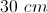long and for every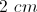there's a tick mark. How many tick marks are there on the ruler?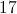Explanation:

There will be 15 gaps oflong but 16 tick marks because there will be a tick mark on each end of the ruler.

### Example Question #7 : How To Find The Number Of Integers Between Two Other Integers

How many prime numbers are between 1 to 25?Explanation:

A prime numbers is a number greater than 1 that can only be divided by 1 and itself. Simply count from 1 to 25 and see how many values fit the criteria.

2, 34, 56, 78, 9, 10, 1112, 1314, 15, 16, 1718, 19, 20, 21, 22, 2324, 25

Prime numbers are underlined. Nine prime numbers are in this set interval.

### Example Question #2 : How To Find The Number Of Integers Between Two Other Integers

Four consecutive odd integers sum to 40. How many of these numbers are prime?Explanation:

Let x equal the smallest of the four numbers. Therefore: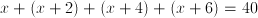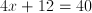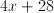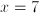Therefore the four odd numbers are 7, 9, 11, and 13. Since all are prime except 9, three of the numbers are prime.

### Example Question #1 : How To Find The Number Of Integers Between Two Other Integers

Find the median of this set of numbers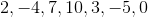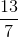Explanation:

To find the median of a set of numbers, we must first order them from smallest to greatest.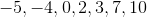Next, we must find the number directly in the middle, which has an equal number of numbers to the right and to the left.

Therefore, our answer is.